×

Search anything:

# Text classification using CNN

#### Machine Learning (ML)Get this book -> Problems on Array: For Interviews and Competitive Programming

Reading time: 40 minutes | Coding time: 15 minutes

In this article, we are going to do text classification on IMDB data-set using Convolutional Neural Networks(CNN). We will go through the basics of Convolutional Neural Networks and how it can be used with text for classification.

• Basics of Convolutional Neural Networks
• How to use CNN for text classification?
• Code Demonstration

# Convolutional Neural Networks

Let's first understand the term neural networks. In a neural network, where neurons are fed inputs which then neurons consider the weighted sum over them and pass it by an activation function and passes out the output to next neuron.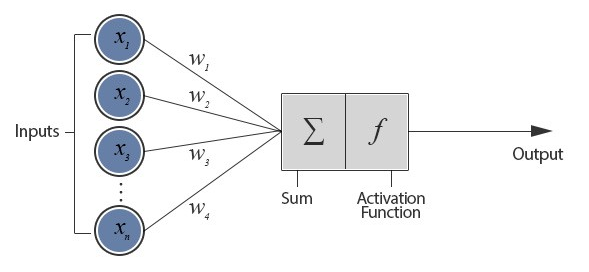Now, a convolutional neural network is different from that of a neural network because it operates over a volume of inputs.

Each layer tries to find a pattern or useful information of the data.

An example of multi-channel input is that of an image where the pixels are the input vector and RGB are the 3 input channels representing channel.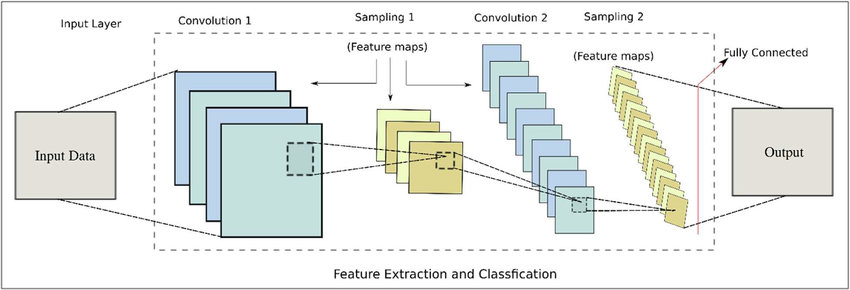This is what the architecture of a CNN normally looks like. It will be different depending on the task and data-set we work on. There are some terms in the architecutre of a convolutional neural networks that we need to understand before proceeding with our task of text classification.

Convolution: It is a mathematical combination of two relationships to produce a third relationship. Joins two sets of information.

Convolution over input: We slide over input data the convolution to extract features by applying a filter/ kernel (both can be used interchangeably). This is important in feature extraction. There are some parameters associated with that sliding filter like how much input to take at once and by what extent should input be overlapped.

• Stride: Size of the step filter moves every instance of time.
• Filter count: Number of filters we want to use.

When we are done applying the filter over input and have generated multiple feature maps, an activation function is passed over the output to provide a non-linear relationship for our output.

An example of activation function can be ReLu.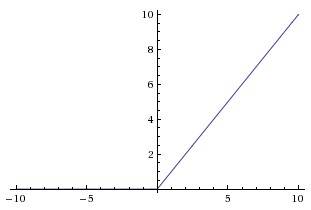Now, we generally add padding surrounding input so that feature map doesn't shrink. If we don't add padding then those feature maps which will be over number of input elements will start shrinking and the useful information over the boundaries start getting lost.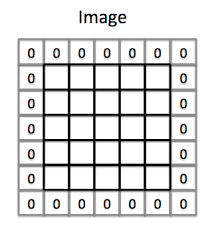It also improves the performance by making sure that filter size and stride fits in the input well.

We are not done yet. We need something that helps us to reduce this high computation in the CNN and not overfit the data. Overfitting will lead the model to memorize the training data rather than learning from it.

We use a pooling layer in between the convolutional layers that reduces the dimensional complexity and stil keeps the significant information of the convolutions.

One example is of max pooling layer. It finds the maximum of the pool and sends it to the next layer as we can see in the figure below.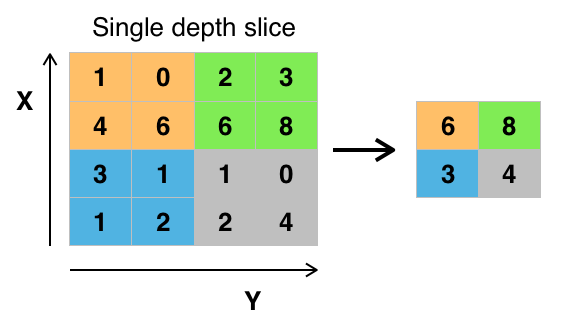Sometimes a Flatten layer is used to convert 3-D data into 1-D vector.

In a CNN, the last layers are fully connected layers i.e. each node of one layer is connected to each node of the other layer.

# How to use for text classification?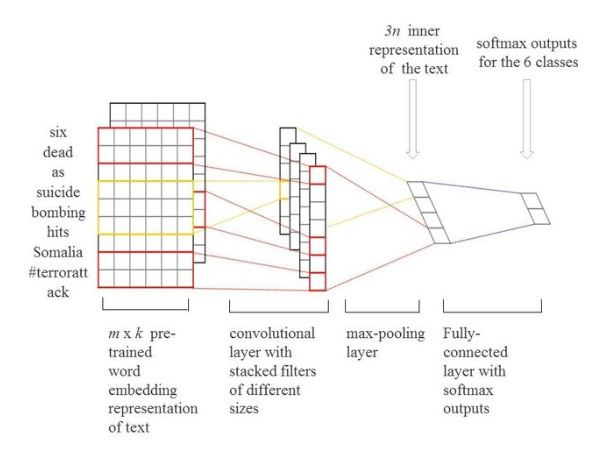A simple CNN architecture for classifying texts

Let's first talk about the word embeddings. When using Naive Bayes and KNN we used to represent our text as a vector and ran the algorithm on that vector but we need to consider similarity of words in different reviews because that will help us to look at the review as a whole and instead of focusing on impact of every single word.

We use a pre-defined word embedding available from the library. Generally, if the data is not embedded then there are many various embeddings available open-source like Glove and Word2Vec.

When we do dot product of vectors representing text, they might turn out zero even when they belong to same class but if you do dot product of those embedded word vectors to find similarity between them then you will be able to find the interrelation of words for a specific class.

Then, we slide the filter/ kernel over these embeddings to find convolutions and these are further dimensionally reduced in order to reduce complexity and computation by the Max Pooling layer.

Lastly, we have the fully connected layers and the activation function on the outputs that will give values for each class.

## Setup

• Python: To run our script
• Pip: Necessary to install Python packages

Now we can install some packages using pip, open your terminal and type these out.

``````pip install tensorflow
pip install keras
``````

Tensorflow: open-source software library for dataflow and differentiable programming across a range of tasks.
Keras: open-source neural-network library

### Code

Let's first start by importing the necessary libraries and the Reuters data-set which is availabe in data-sets provided by keras,

``````# Importing libraries
from keras.datasets import imdb
from keras.models import Sequential
from keras.layers import Dense
from keras.layers import Flatten
from keras.layers.convolutional import Conv1D
from keras.layers.convolutional import MaxPooling1D
from keras.layers.embeddings import Embedding
from keras.preprocessing import sequence
``````
``````# Our dictionary will contain only of the top 7000 words appearing most frequently
top_words = 7000

# Now we split our data-set into training and test data
(X_train, y_train), (X_test, y_test) = imdb.load_data(num_words=top_words)

# Looking at the nature of training data
print(X_train)
print(y_train)

print('Shape of training data: ')
print(X_train.shape)
print(y_train.shape)

print('Shape of test data: ')
print(X_test.shape)
print(y_test.shape)
``````

Output

``````[1, 14, 22, 16, 43, 530, 973, 1622, 1385, 65, 458, 4468, 66, 3941, 4, 173, 36, 256, 5, 25, 100, 43, 838, 112, 50, 670, 2, 9, 35, 480, 284, 5, 150, 4, 172, 112, 167, 2, 336, 385, 39, 4, 172, 4536, 1111, 17, 546, 38, 13, 447, 4, 192, 50, 16, 6, 147, 2025, 19, 14, 22, 4, 1920, 4613, 469, 4, 22, 71, 87, 12, 16, 43, 530, 38, 76, 15, 13, 1247, 4, 22, 17, 515, 17, 12, 16, 626, 18, 2, 5, 62, 386, 12, 8, 316, 8, 106, 5, 4, 2223, 5244, 16, 480, 66, 3785, 33, 4, 130, 12, 16, 38, 619, 5, 25, 124, 51, 36, 135, 48, 25, 1415, 33, 6, 22, 12, 215, 28, 77, 52, 5, 14, 407, 16, 82, 2, 8, 4, 107, 117, 5952, 15, 256, 4, 2, 7, 3766, 5, 723, 36, 71, 43, 530, 476, 26, 400, 317, 46, 7, 4, 2, 1029, 13, 104, 88, 4, 381, 15, 297, 98, 32, 2071, 56, 26, 141, 6, 194, 2, 18, 4, 226, 22, 21, 134, 476, 26, 480, 5, 144, 30, 5535, 18, 51, 36, 28, 224, 92, 25, 104, 4, 226, 65, 16, 38, 1334, 88, 12, 16, 283, 5, 16, 4472, 113, 103, 32, 15, 16, 5345, 19, 178, 32]
1
Shape of training data:
(25000,)
(25000,)
Shape of test data:
(25000,)
(25000,)
``````
• As we see, our dataset consists of 25,000 training samples and 25,000 test samples. Every data is a vector of text indexed within the limit of top words which we defined as 7000 above.

• Now, we pad our input data so the kernel filter and stride can fit in input well. We limit the padding of each review input to 450 words. Keras provides us with function to pad sequences. So, we use it on our reviews.

``````# Padding the data samples to a maximum review length in words
max_words = 450

``````
``````
# Building the CNN Model
model = Sequential()      # initilaizing the Sequential nature for CNN model

# Adding the embedding layer which will take in maximum of 450 words as input and provide a 32 dimensional output of those words which belong in the top_words dictionary

``````
• The model first consists of embedding layer in which we will find the embeddings of the top 7000 words into a 32 dimensional embedding and the input we can take in is defined as the maximum length of a review allowed.

• Then, we add the convolutional layer and max-pooling layer. Finally, we flatten those matrices into vectors and add dense layers(basically scale,rotating and transform the vector by multiplying Matrix and vector).

• The last Dense layer is having one as parameter because we are doing a binary classification and so we need only one output node in our vector.

``````model.compile(loss='binary_crossentropy', optimizer='adam', metrics=['accuracy'])
model.summary()
``````

#### Output

``````Model: "sequential_8"
_________________________________________________________________
Layer (type)                 Output Shape              Param #
=================================================================
embedding_3 (Embedding)      (None, 450, 32)           224000
_________________________________________________________________
conv1d_2 (Conv1D)            (None, 450, 32)           3104
_________________________________________________________________
max_pooling1d_2 (MaxPooling1 (None, 225, 32)           0
_________________________________________________________________
flatten_3 (Flatten)          (None, 7200)              0
_________________________________________________________________
dense_15 (Dense)             (None, 250)               1800250
_________________________________________________________________
dense_16 (Dense)             (None, 1)                 251
=================================================================
Total params: 2,027,605
Trainable params: 2,027,605
Non-trainable params: 0
_________________________________________________________________
``````

Now, we will fit our training data and define the the epochs(number of passes through dataset) and batch size(nunmber of samples processed before updating the model) for our learning model.

• Batch size is kept greater than or equal to 1 and less than the number of samples in training data.
``````# Fitting the data onto model
model.fit(X_train, y_train, validation_data=(X_test, y_test), epochs=2, batch_size=128, verbose=2)

# Getting score metrics from our model
scores = model.evaluate(X_test, y_test, verbose=0)

# Displays the accuracy of correct sentiment prediction over test data
print("Accuracy: %.2f%%" % (scores*100))
``````

#### Output

``````
Train on 25000 samples, validate on 25000 samples
Epoch 1/2
- 28s - loss: 0.4582 - acc: 0.7563 - val_loss: 0.2889 - val_acc: 0.8786
Epoch 2/2
- 27s - loss: 0.2095 - acc: 0.9188 - val_loss: 0.2725 - val_acc: 0.8864
Accuracy: 88.64%
``````

We were able to achieve an accuracy of 88.6% over IMDB movie reviews' test data.

## Tips

• We can improve our CNN model by adding more layers. It is always preferred to have more(dense) layers than to have wide layers of less number.
• But, we must take care to not overfit the data and for that we can try using various regularization methods.#### Harshiv Patel

I’m a junior U.G. pursuing B.Tech Information and Communication Technology at SEAS, Ahmadabad University. My interests are in Data science, ML and Algorithms. Peek into private life = Gaming, Football

Text classification using CNN